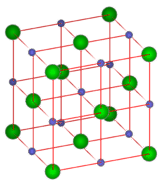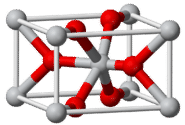# 8.8: Coordination Number

$$\newcommand{\vecs}{\overset { \rightharpoonup} {\mathbf{#1}} }$$ $$\newcommand{\vecd}{\overset{-\!-\!\rightharpoonup}{\vphantom{a}\smash {#1}}}$$$$\newcommand{\id}{\mathrm{id}}$$ $$\newcommand{\Span}{\mathrm{span}}$$ $$\newcommand{\kernel}{\mathrm{null}\,}$$ $$\newcommand{\range}{\mathrm{range}\,}$$ $$\newcommand{\RealPart}{\mathrm{Re}}$$ $$\newcommand{\ImaginaryPart}{\mathrm{Im}}$$ $$\newcommand{\Argument}{\mathrm{Arg}}$$ $$\newcommand{\norm}{\| #1 \|}$$ $$\newcommand{\inner}{\langle #1, #2 \rangle}$$ $$\newcommand{\Span}{\mathrm{span}}$$ $$\newcommand{\id}{\mathrm{id}}$$ $$\newcommand{\Span}{\mathrm{span}}$$ $$\newcommand{\kernel}{\mathrm{null}\,}$$ $$\newcommand{\range}{\mathrm{range}\,}$$ $$\newcommand{\RealPart}{\mathrm{Re}}$$ $$\newcommand{\ImaginaryPart}{\mathrm{Im}}$$ $$\newcommand{\Argument}{\mathrm{Arg}}$$ $$\newcommand{\norm}{\| #1 \|}$$ $$\newcommand{\inner}{\langle #1, #2 \rangle}$$ $$\newcommand{\Span}{\mathrm{span}}$$$$\newcommand{\AA}{\unicode[.8,0]{x212B}}$$Figure $$\PageIndex{1}$$ (Credit: Martin Walker (Wikimedia: Walkerma); Source: Anhydrous: http://commons.wikimedia.org/wiki/File:Cobalt%2528II%2529_chloride.jpg(opens in new window); Hydrated: http://commons.wikimedia.org/wiki/File:Cobalt%2528II%2529_chloride_hexahydrate.jpg(opens in new window); License: Public Domain)

### What makes the colors different?

Cobalt salts contain $$\ce{Co^{2+}}$$ cations. Differences in the color of the salts is due to the species surrounding the cobalt ion. The presence of water molecules in the coordination sphere around the central cobalt ion changes the distances among species and the color of the material.

## Coordination Number

The coordination number is the number of ions that immediately surround an ion of the opposite charge within a crystal lattice. If you examine the figure below, you will see that there are six chloride ions immediately surrounding a single sodium ion. The coordination number of sodium is 6. Likewise, six sodium ions immediately surround each chloride ion, making the coordination number of chloride also equal to 6. Because the formula unit of sodium chloride displays a 1:1 ratio between the ions, the coordination numbers must be the same.Figure $$\PageIndex{2}$$: Lattice structure for sodium chloride. The blue balls represent the sodium ions and the green balls represent the chloride ions. (Credit: User:Eloy/Wikimedia Commons; Source: http://commons.wikimedia.org/wiki/File:NaCl-estructura_cristalina.svg(opens in new window); License: Public Domain)

The formula unit for cesium chloride is $$\ce{CsCl}$$, also a 1:1 ratio. However, as shown in the figure below, the coordination numbers are not 6 as in $$\ce{NaCl}$$. The center ion is the $$\ce{Cs^+}$$ ion and is surrounded by the eight $$\ce{Cl^-}$$ ions at the corners of the cube. Each $$\ce{Cl^-}$$ ion is also surrounded by eight $$\ce{Cs^+}$$ ions. The coordination numbers in this type of crystal are both 8. $$\ce{CsCl}$$ and $$\ce{NaCl}$$ do not adopt identical crystal packing arrangements because the $$\ce{Cs^+}$$ is considerably larger than the $$\ce{Na^+}$$ ion.Figure $$\PageIndex{3}$$: In a cesium chloride crystal, the cesium ion (orange) occupies the center, while the chloride ions (green) occupy each corner of the cube. The coordination number for both ions is 8. (Credit: Christopher Auyeung; Source: CK-12 Foundation; License: CC BY-NC-SA 3.0(opens in new window))

Another type of crystal is illustrated by titanium (IV) oxide, $$\ce{TiO_2}$$, which is commonly known as rutile. The rutile crystal is shown below.Figure $$\PageIndex{4}$$: Titanium (IV) oxide forms tetragonal crystals. The coordination number of the $$\ce{Ti^{4+}}$$ ions (gray) is 6, while the coordination number of the $$\ce{O^{2-}}$$ ions (red) is 3. (Credit: Ben Mills (Wikimedia: Benjah-bmm27; Source: http://commons.wikimedia.org/wiki/File:Rutile-unit-cell-3D-balls.png(opens in new window); License: Public Domain)

The gray $$\ce{Ti^{4+}}$$ ions are surrounded by six red $$\ce{O^{2-}}$$ ions. The $$\ce{O^{2-}}$$ ions are surrounded by three $$\ce{Ti^{4+}}$$ ions. The coordination of the titanium (IV) cation is 6, which is twice the coordination number of the oxide anion, which is 3. This fits with the formula unit of $$\ce{TiO_2}$$, since there are twice as many $$\ce{O^{2-}}$$ ions as $$\ce{Ti^{4+}}$$ ions.

The crystal structure of all ionic compounds must reflect the formula unit. In a crystal of iron (III) chloride, $$\ce{FeCl_3}$$, there are three times as many chloride ions as iron (III) ions.Figure $$\PageIndex{5}$$: Iron (III) chloride. The bluish-gray $$\ce{Fe^{3+}}$$ ions are surrounded by green $$\ce{Cl^-}$$ ions. (CK-12 Foundation)

## Summary

• The coordination number of a compound is determined by the type and number of ions or other species surrounding a central ion.
• Often the color of a compound is affected by the specific materials coordinated to that central ion.

## Review

1. What is the coordination number for Na+ in NaCl?
2. What is the coordination number for Cs+?
3. Why are the packing arrangements for Na+ and Cs+ different?

This page titled 8.8: Coordination Number is shared under a CK-12 license and was authored, remixed, and/or curated by CK-12 Foundation via source content that was edited to the style and standards of the LibreTexts platform; a detailed edit history is available upon request.